# Kerala Syllabus 8th Standard Maths Solutions Chapter 8 Area of Quadrilaterals

## Kerala Syllabus 8th Standard Maths Solutions Chapter 8 Area of Quadrilaterals

Textbook Page No 149

Area Of Quadrilateral Class 8 Scert Chapter 8 Question 1.
Draw a parallelogram of sides 5 centimetres, 6 centimetres and area 25 square centimetres.
Solution:

• Area of parallelogram = one side × distance to the opposite side.
• Since 5x distance to the opposite side is 25 sq.cm. Distance to the opposite side is 5 cm.
• Draw a square ABCD of side 5cm.
• Draw an arc of radius 6cm with centre A to cut DC at E.
• Length of radius 6cm with centre B and arc of radius 5 cm with centre E meet at F.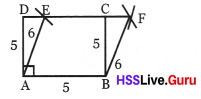Class 8 Mathematics Area Of Quadrilaterals Kerala Scert Solutions Question 2.
Draw a parallelogram of area 25 square centimetres and perimeter 24 centimetres.
Solution:
Area of the parallelogram to be 25 cm2. The product of one side and distance to the opposite side to be 25 cm2.
One side and distance to the opposite side can be 5 cm each.
The other side is = 12 – 5 = 7 cm.
Draw a square with side cm and draw an arc of radius 7 cm with A as centre such that it intersects the side CD. Mark the point E and join AE. Draw an arc of radius 7 cm with B as centre and draw another arc of radius 5 cm with E as centre, Two arcs meet at the point
P. JoinBF and CF.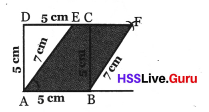Area Of Quadrilaterals Class 8 Kerala Syllabus Question 3.
In the figure, the two bottom corners of a parallelogram are joined to a point on the top side.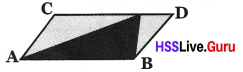Solution:
Area of dark triangle = $$\frac{1}{2} b h$$ cm2
Area of parallelogram = bh
= 2 × area of triangle 10 cm2

Class 8 Mathematics Construction Of Quadrilaterals Kerala Scert Solutions Question 4.
The picture below shows the parallelogram formed by the intersection of two pairs of parallel lines?What is the area of this parallelogram? And the perimeter?
Solution:
One side of the parallelogram = 4 cm
Distance to the opposite side = 3 cm
Area of the parallelogram = 4 × 3 = 12 sq.cm
BC × DE = 12
BC × 2 = 12
BC = 6, AD = 6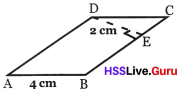Perimeter = 4 + 6 + 4 + 6 = 20 cm.

Area Of Quadrilateral Questions For Class 8 Kerala Syllabus Question 5.
Compute the area of the parallelogram below:Solution: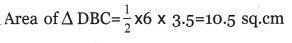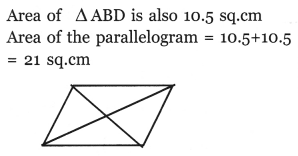Textbook Page No 153

Hss Live Guru 8 Maths Kerala Syllabus Chapter 8 Question 6.
Draw a square of area 4½ square centimetres.
Solution: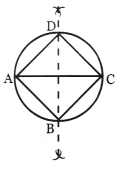Draw a circle of radius 3 cm. Draw the diameter AC and construct the perpendicular bisector of AC which meets the circle at B and D. Join AC, AD, CD and BD to get the square ABCD.

Area Of Quadrilateral Formula Class 8 Kerala Syllabus  Question 7.
Draw a non-square rhombus of area 9 square centimetres.
Solution:• Draw AC = 1 cm
• Find midpoint of AC by drawing per perpendicular bisector
• Find B and D, such that OB = OD = 9 cm

Similarly we can draw the other two rhombus
AC = 9 cm,
OB = OD = 1 cm
AC = 3 cm, OD = OB = 3 cm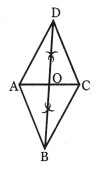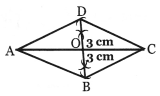8th Standard Maths Notes Kerala Syllabus Chapter 8 Question 8.
The area of the dark triangle in the figure is 5 square centimetres. What is the area of the parallelogram?
Solution:iii. Perimeter = 4 × 15 = 60 m
iv. Rhombus is a parallelogram. So,
Area of parallelogram = one side × distance to the opposite side
15 × h = 216
h = $$\frac{216}{15}=14.4 \mathrm{cm}$$

8th Standard Maths Notes PdfKerala Syllabus Chapter 8 Question 9.
A 68 centimetre long rope is used to make a rhombus on the ground. The distance between a pair of opposite corners is 16 metres.
i. What is the distance between the other two corners?
ii. What is the area of the ground bounded by the rope?
Solution:Length of one side of a parallelogram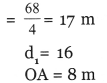Diagonals are perpendicular bisectorKerala Syllabus 8th Standard Maths Notes Pdf Chapter 8 Question 10.
In the figure, the midpoints of the diagonals of a rhombus are joined to form a small quadrilateral:i. Prove that this quadrilateral is a rhombus.
ii.The area of the small rhombus is 3 square centimetres. What is the area of the large rhombus?
Solution: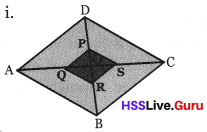The diagonals of the rhombus intersect at O and they bisect each other at right angles.
OA = OC
$$\frac{\mathrm{OA}}{2}=\frac{\mathrm{OC}}{2}$$
OQ = OS
Similarly, since OB = OD
ie., OR = OP
The diagonals of PQRS bisect each other. (Since OQ = OS and OR = OP)
Since the diagonal AC is perpendicular to BD, the diagonals of PQRS are also mutually perpendicular bisectors.
therefore PQRS is a rhombus

ii. Area of quadrilateral PQRS = 3 sq. cm.8th Area Of Quadrilateral Formula Class 8 Kerala Syllabus Question 11.
What is the area of the largest rhombus that can be drawn inside a rectangle of sides 6 centimetres and 4 centimetres?
Solution:
If we join the midpoints of the opposite sides of rectangle, we get the longest diagonal of the rhombus.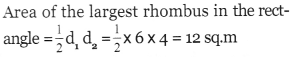Textbook Page No 156

Hsslive 8th Maths Kerala Syllabus Chapter 8 Question 12.
Draw a rectangle of sides 7 centimetres and 4 centimetres. Draw isosceles trapeziums of the same area, with the following specifications.
i. Lengths of parallel sides 9 centimetres, 5 centimetres.
ii. Lengths of non-parallel sides 5 centimetres.
Solution:
i. Draw a rectangle of length 7 cm and breadth 4 cm.Extent AB either side by 1 cm and mark E and F. Also mark the points G and H on CD at a distance 1 cm from C and D respectively. Draw the rhombus EFGH.ii. Draw the rectangle ABCD.The arc of radius 5cm drawn with A as centre intersects DC at P. The arc of 5cm drawn with C as the centre intersect extented AB at Q.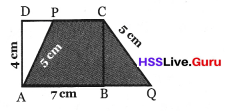Std 8 Maths Kerala Syllabus Kerala Syllabus Chapter 8 Question 13.
Calculate the area of the isosceles trapezium drawn below: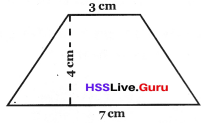Solution: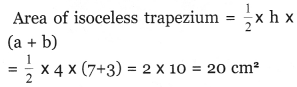8th Std Maths Notes Kerala Syllabus Chapter 8 Question 14.
The parallel sides of an isosceles trapezium are 8 centimetres and 4 centimetres long; and non – parallel sides are 5 centimetre long. What is its area?
Solution:
Divide the isosceles trapezium into a rectangle and two triangles.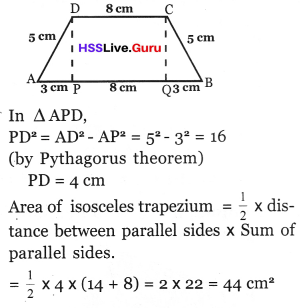Textbook Page No 158

Area Of Quadrilateral Class 8 Kerala Syllabus Chapter 8 Question 15.
The lengths of the parallel sides of a trapezium are 30 centimetres, 10 centimetres and the distance between them is 20 centimetres. What is its area?
Solution:Question 16.
Compute the area of the trapezium shown below: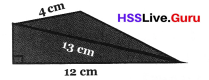Solution:
Consider the ∆ PQS, it is a right angled triangle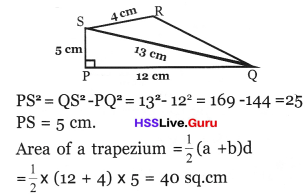Textbook Page No 159

Question 17.
Compute the area of the hexagon below.Solution: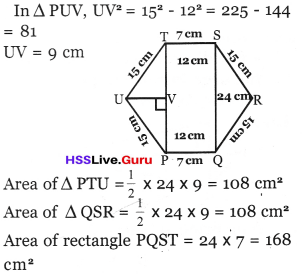Total area = 108 + 108 + 168 = 384 cm2

Question 18.
This is a picture drawn in the lesson Construction of Quadrilaterals.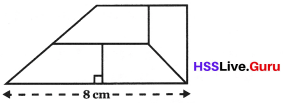What is the area of the large trapezium made up of the four smaller ones?
Solution:Question 19.
What is the area of the quadrilateral shown below?Solution:Question 20.
Prove that for any quadrilateral with diagonals perpendicular, the area is half the product of the diagonals.
Solution:Question 21.
Compute the area of the quadrilateral shown below: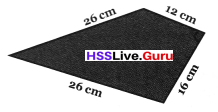Solution: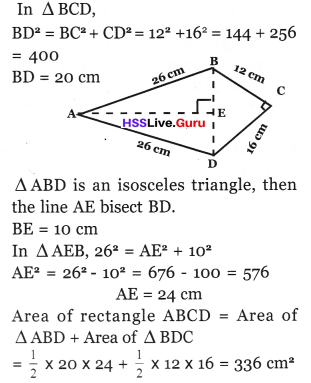Question 22.
Compute the area of the parallelogram shown below: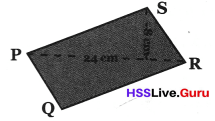Solution:
The diagonal divides the parallelogram into two equal triangles.Area of the parallelogram = 2 × area of triangle = 2 × 96 = 192 cm2

Question 23.
The three blue lines in the picture below are parallel:Prove that the areas of the quadrilaterals ABCD and PQRS are in the ratio of the lengths of the diagonals AC and PR.
i. How should the diagonals be related for the quadrilaterals to have equal area?
ii. Draw two quadrilaterals, neither parallelograms nor trapeziums, of area 15 square centimetres
Solution: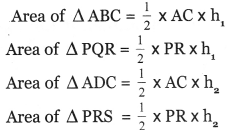i. For the quadrilaterals to have equal area, the diagonals AC and PR should be equal.
ii. Draw three parallel lines, distance between them is 5 cm each. Also draw two quadrilaterals with diagonals PQ and RS 6 cm each.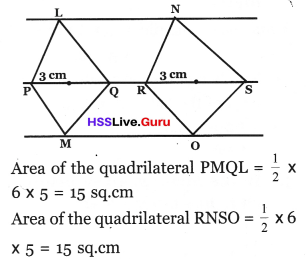Question 1.
Which of the following has greater area; a parallelogram with one side 12 cm and distance between parallel sides is 6 cm or a square having diagonals 12 cm each?
Solution:Question 2.
Area of a trapezium is 128 sq. cm and the distance between its parallel sides is 8 cm. Length of one parallel side is 28 cm. Find the length of the other parallel side.
Solution: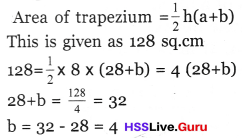Length of the other Parallel side = 4 cm

Question 3.
Find the area of a rhombus of one side 6 cm and one diagonal 6 cm.
Solution: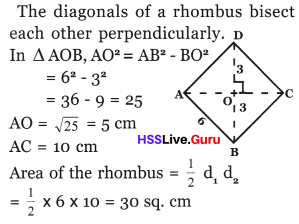Question 4.
It was decided to lay tiles in the room of Hari’s house with tiles in the shape of trapezium. The parallel sides of a tile is 40 cm and 20 cm and the distance between them is 10 cm. If 200 tiles are needed to lay tiles in the hall, find the area of the hall in square metres.
Solution: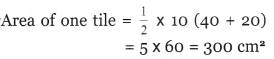Number of tiles required = 200
Area of the hall = 200 × 300
= 60000 cm2
= 6 m2

Question 5.
In the pictures given below, which is has more area?Solution:
Rectangle has the maximum area among the parallelograms with same sides.

Question 6.
The ratio of the two adjacent sides of a parallelogram is 3 : 2. Distance between longer sides is 10 cm. If area is 900 cm2, find the sides of the parallelogram?
Solution:
Let the sides be 3x and 2x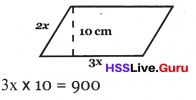Question 7.
If one side of a parallelogram is ‘a’ and height of that side is h, prove that area = ah.
Solution:
In the figure, ABCD is a parallelogram. AB = a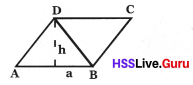The perpendicular distance from D to AB = h
By drawing the diagonal BD, we can divide the parallelogram into two equal triangles. ∆ ABD and ∆ BCD are equal triangles. ∴ their areas are equal, ie; area of the parallelogram is two times of the area of ∆ ABD.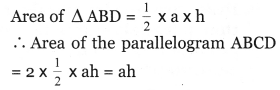Question 8.
PQRS is a rhombus. If the diagonals are 8 cm and 9 cm each, compute the area.Solution:
Let the diagonals of the rhombus are d1 and d2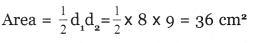Question 9.
The perimeter of a rhombus is 40 cm. If the length of one diagonal is 16 cm. What is the length of the other diagonal. Find the area?
Solution:
Perimeter = 40 cm
One side = 10 cm .
∆ POQ is a right angled triangle Since d1 = 16 cm, OP = 8 cm
∴ 82 + OQ2 = 102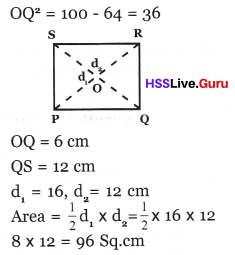Question 10.
Draw a square of area 12.5 cm2 and write the geometric principles of the construction.
Solution: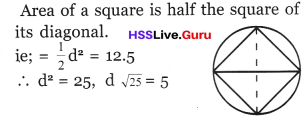Draw a line of length 5 cm and its perpendicular bisector.
Draw a circle with the midpoint of this line as centre and the distance to one end as radius. This line will be the diameter of the circle. Mark the point of intersection of the perpendicular bisector as the circle and complete the square.

Question 11.
Compute the area of the trapezium shown below.Solution: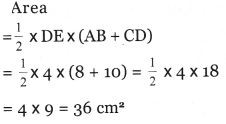Question 12.
Compute the area of the quadrilateral ABCD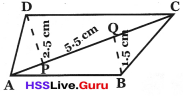Solution: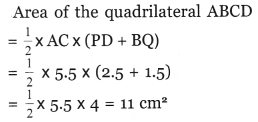Question 13.
In the figure AB || CD. AD = BC = 13 cm
Distance between the parallel side is 12 cm. If CD = 20 cm. Find the area of ABCD.Solution:
To compute AB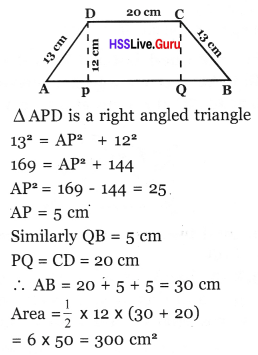Question 14.
Draw a square and mark the mid-points. How is the area of the first square with the second square.
Solution:
Draw the square ABCD.
The perpendicular bisector of the side AB bisects CD also at right angles.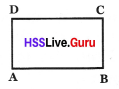Similarly draw the perpendicular bisectors of AD and BC. Which intersect the sides. Join the points of intersection to complete the square.We see, 8 triangles in the figure. The square PQRS is formed by joining 4 among these. The area of the square PQRS is half of the area of the square ABCD.

Question 15.
What is the maximum area of parallelogram of sides 8 cm and 5 cm? What is the speciality of the parallelogram of maximum area?
Solution:
The area will be maximum for a rect¬angle and the maximum area is 40 cm2.

Question 16.
See the quadrilateral Ravi drew. In it AC = 12 cm and perpendiculars to AC are 6 cm and 12 cm.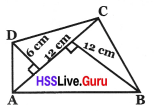a. Find the area of the quadrilateral.
b. Raju has to draw a parallelogram of this area. If its base is 12 cm what should be its height?
Solution:b. If the height of the parallelogram is h its area is bh = 12 × h
This is 54 sq. cm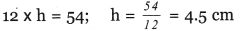Question 17.
The area of a rhombus is 112 sq. cm and one of its diagonal is 16 cm long. Find the length of the other diagonal.
Solution:
Area of the rhombus $$=\frac{1}{2} \mathrm{d}_{1} \times \mathrm{d}_{2}$$
= 112 sq. cmQuestion 18.
Draw a parallelogram of sides 6 cm, 4 cm and area 18 cm2.
Solution:
Draw a line AB of length 6 cm. Draw a circle with centre A and radius 4 cmIn the circle (as shown in the picture) mark a point D and join AD. Draw an arc of radius 4 cm with centre B as centre and radius 4 cm and draw another arc with D as centre and radius 6 cm. Two arcs meet at C. Join CD and BC.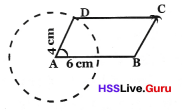ABCD will be a parallelogram.

Question 19.
In the figure ABCD, AB parallel to CD and the distance between them is 8 cm. ?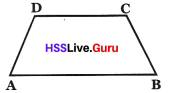AB = 12 cm, CD = 10 cm. Compute the area of quadrilateral (trapezium) ABCD?
Solution:Question 20.
Compute the area of the quadrilateral ABCD in the figure.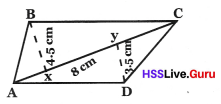Solution: